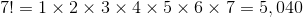# ISEE Upper Level Quantitative : How to find out if a number is prime

## Example Questions

← Previous 1 3 4 5

### Example Question #1 : How To Find Out If A Number Is Prime

Multiply the two greatest prime numbers less than 100.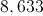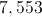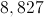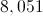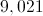Explanation:

The two greatest prime numbers less than 100 are 97 and 89. Their product is: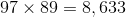### Example Question #2 : How To Find Out If A Number Is Prime

Which of the following numbers has exactly two factors?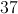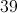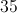None of the other choices is correct.Explanation:

Every number except 1 has at least two factors - 1 and itself. So we are looking for a number with no other factors - that is, a prime.

3 is a factor of both 33 and 39, and 5 is a factor of 35, so we can eliminate those choices. We cannot eliminate 37, since it is a prime, having only 1 and 37 as factors.

### Example Question #3 : How To Find Out If A Number Is Prime

Multiply the two smallest prime numbers greater than 100.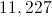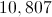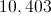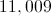Explanation:

The first two primes after 100 are 101 and 103; their product is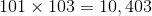.

### Example Question #4 : How To Find Out If A Number Is Prime

Which is the greater quantity?

(a) The number of prime numbers between 70 and 110

(b) The number of prime numbers between 80 and 120

(b) is greater

(a) and (b) are equal

(a) is greater

It is impossible to tell from the information given

(a) is greater

Explanation:

The primes between 80 and 110 are included in both sets, so all we need to do is to compare the number of primes between 70 and 80 and the number of primes between 110 and 120.

(a) The primes between 70 and 80 are 71, 73, and 79 - three primes

(b) The only prime between 110 and 120 is 113.

(a) is the greater quantity

### Example Question #5 : How To Find Out If A Number Is Prime

Which is the greater quantity?

(a) The number of prime numbers between 1 and 20 inclusive

(b) The number of composite numbers between 21 and 30 inclusive

(a) and (b) are equal

It is impossible to tell from the information given

(b) is greater

(a) is greater

(a) and (b) are equal

Explanation:

(a) The prime numbers between 1 and 20 inclusive are 2, 3, 5, 7, 11, 13, 17, 19 - eight total.

(b) The prime numbers between 21 and 30 inclusive are 23 and 29 - two prime numbers out of ten integers. This leaves eight composite numbers.

(a) and (b) are therefore equal.

### Example Question #6 : How To Find Out If A Number Is Prime

Which is the greater quantity?

(a) The number of prime numbers between 1 and 20 inclusive

(b) The number of composite numbers between 1 and 20 inclusive

(b) is greater

It is impossible to tell from the information given

(a) and (b) are equal

(a) is greater

(b) is greater

Explanation:

The prime numbers between 1 and 20 inclusive are 2, 3, 5, 7, 11, 13, 17, 19 - eight total. Since 1 is neither prime nor composite, this leaves 11 composite numbers. (b) is the greater quantity.

### Example Question #7 : How To Find Out If A Number Is Prime

Which is the greater quantity?

(a) The sum of the prime numbers between 11 and 19 inclusive

(b) The sum of the composite numbers between 11 and 19 inclusive

(a) and (b) are equal

(b) is the greater quantity

(a) is the greater quantity

It is impossible to tell from the information given

(b) is the greater quantity

Explanation:

(a) The prime numbers in the 11-19 range are 11, 13, 17, and 19. Their sum: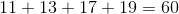(b) The composite numbers in the 11-19 range are 12, 14, 15, 16, and 18. Their sum: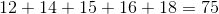(b) is greater.

### Example Question #8 : How To Find Out If A Number Is Prime

Which quantity is greater?

(a) The number of composite numbers between 1 and 1,000 inclusive.

(b) The number of even integers between 1 and 1,000 inclusive.

It is impossible to tell from the information given

(a) and (b) are equal

(a) is greater

(b) is greater

(a) is greater

Explanation:

It is not necessary to count all of the composite numbers in the 1-1,000 range. Except for 2, every even number is composite, and there are more than one odd composite numbers (15, 25) to make up for 2. This makes (a) greater.

### Example Question #9 : How To Find Out If A Number Is Prime

Which is the greater quantity?

(a) The number of primes between 20 and 50

(b) The number of primes between 10 and 40

(b) is greater.

(a) and (b) are equal.

(a) is greater.

It is impossible to tell from the information given.

(b) is greater.

Explanation:

The primes between 20 and 40 are included in both sets, so all we need to do is to compare the number of primes between 40 and 50 with the number of primes between 10 and 20.

(a) The primes between 40 and 50 are 41, 43, and 47 - three.

(b) The primes between 10 and 20 are 11, 13, 17, and 19 - four.

Since the number of primes between 10 and 20 outnumbers those between 40 and 50, the number of primes between 10 and 40 outnumber those between 20 and 50. Therefore, (b) is the greater.

### Example Question #10 : How To Find Out If A Number Is Prime

Which is the greater quantity?

(a) The product of the first five prime numbers

(b)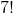It is impossible to tell from the information given.

(b) is greater.

(a) is greater.

(a) and (b) are equal.

(a) The product of the first five prime numbers is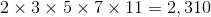.
(b)